Function Repository Resource:

# MapSlice

Provide the part specifications to a mapped function as a sequence of arguments after the first one

Contributed by: Seth J. Chandler
 ResourceFunction["MapSlice"][f,expr] applies f to the elements of expr, giving the sequence of part specifications of each element as the remaining argument(s) to f. ResourceFunction["MapSlice"][f,expr,levelspec] applies f to all parts of expr on levels specified by levelspec, with these part indices provided as the remaining arguments to f. ResourceFunction["MapSlice"][f] represents an operator form of ResourceFunction["MapSlice"] that can be applied to an expression at its first level.

## Details and Options

ResourceFunction["MapSlice"] is a variant of MapIndexed that gives the part specifications as a sequence of arguments rather than a single List argument.
ResourceFunction["MapSlice"] uses standard level specifications just like MapIndexed.
The default value for levelspec in ResourceFunction["MapSlice"] is {1}.
A positive level n consists of all parts of expr specified by n indices.
A negative level -n consists of all parts of expr with depth n.
Level –1 consists of numbers, symbols and other objects that do not have subparts.
Level 0 corresponds to the whole expression.
ResourceFunction["MapSlice"] traverses the parts of expr in a depth-first order, with leaves visited before roots.
ResourceFunction["MapSlice"] always effectively constructs a complete new expression and then evaluates it.
ResourceFunction["MapSlice"] works on SparseArray objects, effectively by applying Normal to them.
ResourceFunction["MapSlice"] works on Association objects, giving part specifications in the form Key[k].
ResourceFunction["MapSlice"][f][expr] is equivalent to ResourceFunction["MapSlice"][f,expr,{1}].

## Examples

### Basic Examples (5)

Apply f to a list with four parts:

 In:=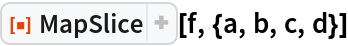Out=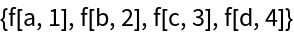Argument #2 gives the index of each part:

 In:=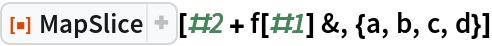Out=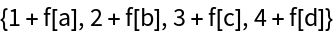Changing the level specification affects the output:

 In:=Out=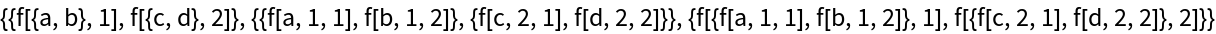Use the operator form of MapSlice:

 In:=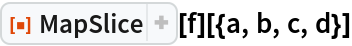Out=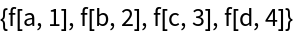Use MapSlice with an association:

 In:=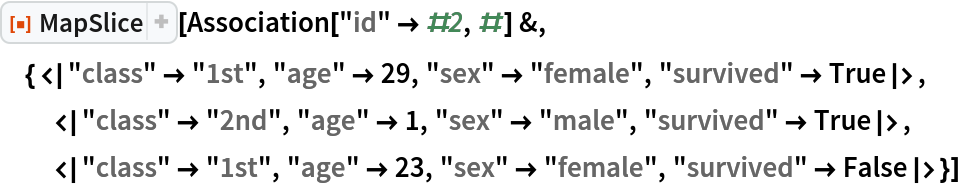Out=### Scope (5)

Functions used inside MapSlice may contain slot values as high as one more than the level specification:

 In:=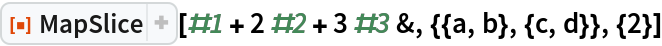Out=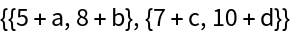Map onto all the elements of an expression:

 In:=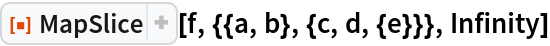Out=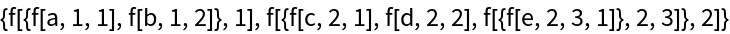Map only onto the "leaves" of the expression:

 In:=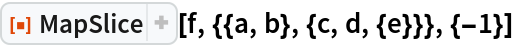Out=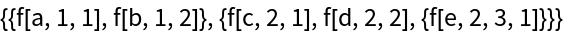Map on levels 0 through 1; the head has index {}:

 In:=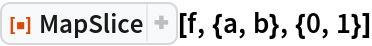Out=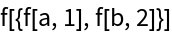MapSlice can be used on expressions with any head:

 In:=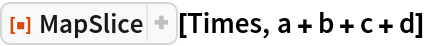Out=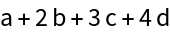### Applications (3)

Label parts by position:

 In:=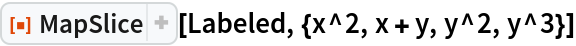Out=In:=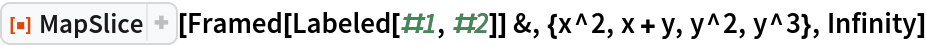Out=Rotate lists based on position:

 In:=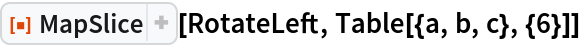Out=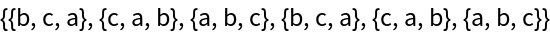Show where various elements of a three-dimensional array might be stored in memory:

 In:=Out=### Properties and Relations (1)

You can emulate MapIndexed using MapSlice:

 In:=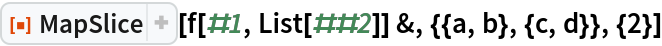Out=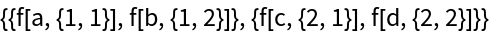### Possible Issues

There is no Heads option to MapSlice as there is for MapIndexed.

### Neat Examples (1)

The following two pieces of code are equivalent, but the version with MapSlice is arguably cleaner because there is no need for a Part function within the first argument; this will often be the case for functions that map at level 1:

 In:=Out=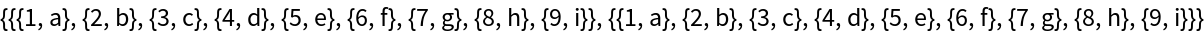Seth J. Chandler

## Version History

• 1.0.0 – 15 July 2019